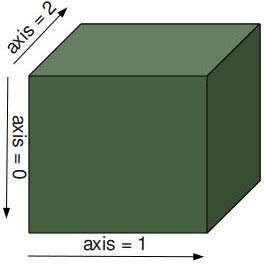riset (90) tutorial (68) python (62) tajuk (35) matlab (27) data minning (7) java (6) C# (5) kriptografi (4) mysql (3) Steganografi (1) buku terbit (1) optimasi (1) pyQT (1) web service (1)

## Jumat, 24 Maret 2017

### How to append vector in numpyThese values are appended to a copy of arr. It must be of the correct shape (the same shape as arr, excluding axis),

``````
import numpy as np

a = np.array([[1,2,3],[4,5,6]])
b = np.array([7,8,9])
c = np.vstack((a,b))
print c

a = np.array([[1,2,3],[4,5,6]])
b = np.array([7,8])
b = b.reshape(np.size(b),1)
c = np.hstack((a,b))
print c ``````